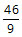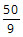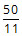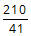# GMAT Practice Questions - Word Problems

Must solve GMAT practice questions in word problems. The following topics are covered in the GMAT quant section from word problems:

1. Speed Time
2. Work Time
3. Ratio and Proportions
4. Mixtures
1. Percents
2. Profits
3. Interest
4. Data Interpretation

Click on the Problem Solving or the Data Sufficiency tab for practice questions. The topic from which the question has been selected and the concept tested are listed in the right column for each question. Detailed explanatory answers, solution videos and slide decks are also provided.

• 1
• Working alone at their respective constant rates, A can complete a task in ‘a’ days and B in ‘b’ days. They take turns in doing the task with each working 2 days at a time. If A starts they finish the task in exactly 10 days. If B starts, they take half a day more. How long does it take to complete the task if they both work together? A.B.C.D.E.• Algebra
• Problem Solving
• Work Time
Working together, they will complete the task in 36/7 days.

Solution

• 1
• A candy manufacturer decided to decrease the weight of each candy bar, while retaining the price. By how many cents did the per kilogram cost of candy change after the reduction in weight? Statement 1: The weight of each piece of candy bar reduced by 9 grams.
Statement 2: The weight of each piece of candy bar reduced by 9%
• Data Sufficiency
• Ratios, Percents, Fractions